Courses

# Test: Methods of Indeterminate Analysis - 3

## 20 Questions MCQ Test Topicwise Question Bank for Civil Engineering | Test: Methods of Indeterminate Analysis - 3

Description
This mock test of Test: Methods of Indeterminate Analysis - 3 for Civil Engineering (CE) helps you for every Civil Engineering (CE) entrance exam. This contains 20 Multiple Choice Questions for Civil Engineering (CE) Test: Methods of Indeterminate Analysis - 3 (mcq) to study with solutions a complete question bank. The solved questions answers in this Test: Methods of Indeterminate Analysis - 3 quiz give you a good mix of easy questions and tough questions. Civil Engineering (CE) students definitely take this Test: Methods of Indeterminate Analysis - 3 exercise for a better result in the exam. You can find other Test: Methods of Indeterminate Analysis - 3 extra questions, long questions & short questions for Civil Engineering (CE) on EduRev as well by searching above.
QUESTION: 1

### Which one of the following statements is correct? The portal method of structural analysis is generally suitable for:

Solution:

Portal method is used for low rise buildings with/without uniform framing.

QUESTION: 2

### Due to settlement of support at A of propped cantilever shown in the figure given below, what is the vertical reaction at B?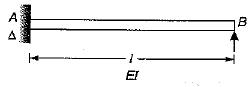Solution: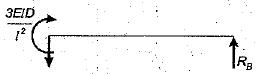Free body diagram of beam is shown in the figure.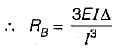QUESTION: 3

### For the beam shown below, what are the distribution factors at joint B?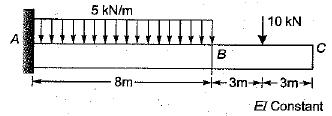Solution:

Stiffness of member BA = 4EI/8 = 0.5 El
Stiffness of member BC = 3EI/6 =  0.5 El
Distribution factors at joint S will be 0.5 , 0.5.

QUESTION: 4

A fixed beam AB with a central hinge C is built of two components. AC is rigid and CB has a moment of inertia I. When support A yields and rotates through θ, what-is the moment at B?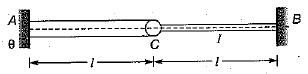Solution:

When support A yields and rotates through θ, the whole beam AC rotates due to its rigidity. The deflection diagram of beam AB is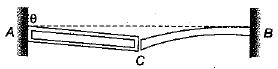The rotation at end C of beam BC is θ,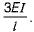Therefore moment at B is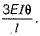QUESTION: 5

For a symmetrical continuous beam as shown in the figure given below, which one of the following is correct in respect of distribution factors at B?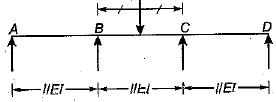Solution:

At joint B.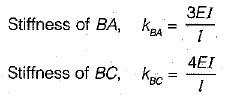The distribution factors.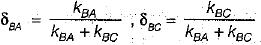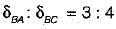QUESTION: 6

What is the horizontal sway of the point A of the portal frame shown in the figure given below?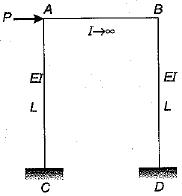Solution:

Let the horizontal sway at A is Δ. So moment at ends A and C of column AC is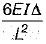and  horizontal shear at C is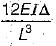horizontal shear at support D is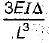From equilibrium of horizontal forces.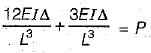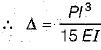QUESTION: 7

What is the fixed end moment for the beam shown in the figure given below?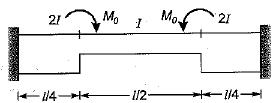Solution:

As the beam and loading are symmetrical so fixed end moments will also be same. From Mohr’s theorem (the end rotations being zero) the area of free and fixed bending moment diagrams will be equal
Free moment diagram,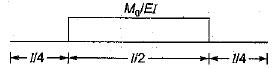Fixed moment diagram,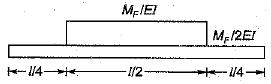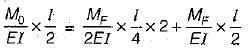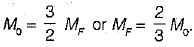QUESTION: 8

Unequal settlements in the supports of a statically determinate structure develop

Solution:

In statically determinate structure, structure develop no reactions on settlement while in Statically in determinate structure , structure develop reactions.

QUESTION: 9

A fixed beam of span L is carrying a point load P at its mid-span. If the moment of inertia of the middle half length is two times that of the remaining length, then the fixed end moments will be

Solution:
QUESTION: 10

A rigid-jointed plane frame as shown below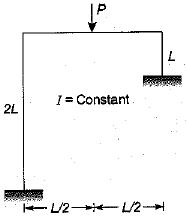Solution:

The frame will sway towards the side which has lesser I/L (relative stiffness).

QUESTION: 11

If a point load acting at the mid-span of a fixed beam of uniform section produces fixed end moments of 60 kN-m, then the same load spread uniformly over the entire span will produce fixed end moments equal to

Solution:

’Fixed end'moment due to central point load,
M1 = PL/8 = 60 kNm
The fixed end moment due to uniformly distributed load is PL/12. Thus it is equal to 40 kN-m.

QUESTION: 12

Consider the following statements:
Hardy Cross method of moment distribution can be applied to analyze
1. Continuous beams including non-prismatic structures
2. Continuous beams with prismatic elements
3. Structures with intermediate hinges
4. Rigid frames

Which of these statements are correct?

Solution:

Hardy cross method or moment distribution method can be applied even when there is intermediate hinges.

QUESTION: 13

A plane frame is loaded as shown in figure. The rotations are indicated as θB and θC and sway is indicated by the symbol Δ. For the given frame which of the following statements is correct?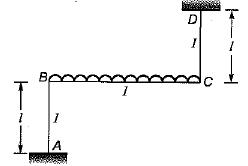Solution:

The structure is symmetrical but loading is anti- symmetrical about mid point of BC. If a symmetric structure is subjected to antisymmetric loading, the structural response is antisymmetric.
θB = -θC; Δ is present

QUESTION: 14

When a load is applied to a structure with rigid joints

Solution:
QUESTION: 15

For the portal frame shown in figure below the final end moments are
MAB = 15 kNm
MBA = 10 kNm
MCD = 20 kNm
The magnitude of end moment at D(MDC) will be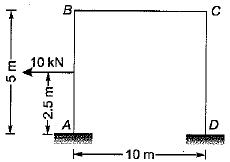Solution:
QUESTION: 16

Bending moment diagram for the structure shown below is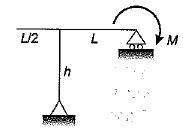Solution: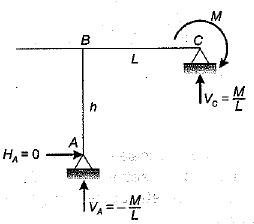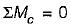⇒ VA x L + M = 0
∴ V= - M/L
AS VA+VC =0
⇒ VL = M/L
For span BC,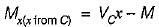∴ Mc = - M
and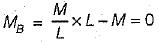QUESTION: 17

The shear equation for the portal frame as shown below is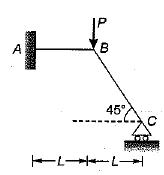Solution: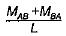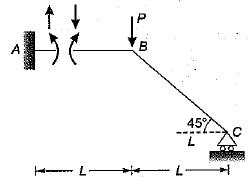Taking moment of ail forces about C,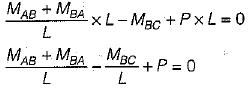QUESTION: 18

Consider the following methods:
1. Slope deflection method
2. Three moment method
3. Column analogy method
4. Kani’s method

Which of the above method(s) is/are the force method of structural analysis

Solution:
QUESTION: 19

A two span continuous beam ABC is simply supported at A and C and is continuous over support B. Span AB = 6 m, BC = 6m. The beam carries a UDL of 2 t/m over both the spans. EI is constant for the entire beam. The fixed end moment at B in span BA or BC would be

Solution:

Using three moment theorem,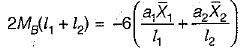as MA = Mc = 0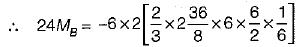or MB = 9 t . m .

QUESTION: 20

The rotation at joint B of portal frame shown in figure for members BA and BC will be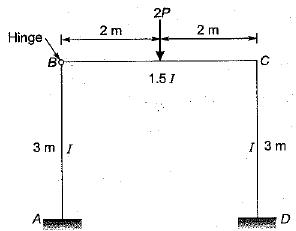Solution:

Rotation at joint B for member BA and BC will be different as flexural rigidity of both members are different.